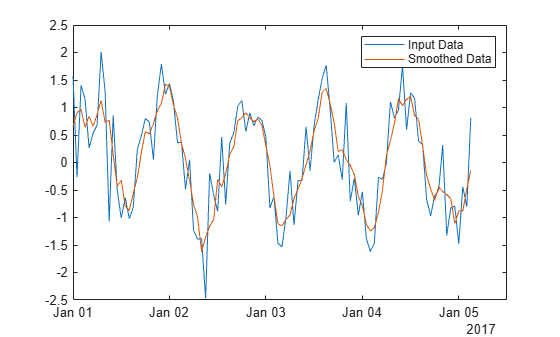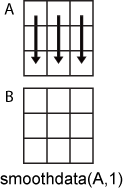Documentation

# smoothdata

Smooth noisy data

## Syntax

``B = smoothdata(A)``
``B = smoothdata(A,dim)``
``B = smoothdata(___,method)``
``B = smoothdata(___,method,window)``
``B = smoothdata(___,nanflag)``
``B = smoothdata(___,Name,Value)``
``````[B,window] = smoothdata(___)``````

## Description

example

````B = smoothdata(A)` returns a moving average of the elements of a vector using a fixed window length that is determined heuristically. The window slides down the length of the vector, computing an average over the elements within each window. If `A` is a matrix, then `smoothdata` computes the moving average down each column.If `A` is a multidimensional array, then `smoothdata` operates along the first dimension whose size does not equal 1.If `A` is a table or timetable with numeric variables, then `smoothdata` operates on each variable separately. ```

example

````B = smoothdata(A,dim)` operates along the dimension `dim` of `A`. For example, if `A` is a matrix, then `smoothdata(A,2)` smooths the data in each row of `A`.```

example

````B = smoothdata(___,method)` specifies the smoothing method for either of the previous syntaxes. For example, ```B = smoothdata(A,'sgolay')``` uses a Savitzky-Golay filter to smooth the data in `A`.```

example

````B = smoothdata(___,method,window)` specifies the length of the window used by the smoothing method. For example, `smoothdata(A,'movmedian',5)` smooths the data in `A` by taking the median over a five-element sliding window.```

example

````B = smoothdata(___,nanflag)` specifies how `NaN` values are treated for any of the previous syntaxes. `'omitnan'` ignores `NaN` values and `'includenan'` includes them when computing within each window.```

example

````B = smoothdata(___,Name,Value)` specifies additional parameters for smoothing using one or more name-value pair arguments. For example, if `t` is a vector of time values, then `smoothdata(A,'SamplePoints',t)` smooths the data in `A` relative to the times in `t`.```

example

``````[B,window] = smoothdata(___)``` also returns the moving window length.```

## Examples

collapse all

Create a vector containing noisy data, and smooth the data with a moving average. Plot the original and smoothed data.

```x = 1:100; A = cos(2*pi*0.05*x+2*pi*rand) + 0.5*randn(1,100); B = smoothdata(A); plot(x,A,'-o',x,B,'-x') legend('Original Data','Smoothed Data')```Create a matrix whose rows represent three noisy signals. Smooth the three signals using a moving average, and plot the smoothed data.

```x = 1:100; s1 = cos(2*pi*0.03*x+2*pi*rand) + 0.5*randn(1,100); s2 = cos(2*pi*0.04*x+2*pi*rand) + 0.4*randn(1,100) + 5; s3 = cos(2*pi*0.05*x+2*pi*rand) + 0.3*randn(1,100) - 5; A = [s1; s2; s3]; B = smoothdata(A,2); plot(x,B(1,:),x,B(2,:),x,B(3,:))```Smooth a vector of noisy data with a Gaussian-weighted moving average filter. Display the window length used by the filter.

```x = 1:100; A = cos(2*pi*0.05*x+2*pi*rand) + 0.5*randn(1,100); [B, window] = smoothdata(A,'gaussian'); window```
```window = 4 ```

Smooth the original data with a larger window of length 20. Plot the smoothed data for both window lengths.

```C = smoothdata(A,'gaussian',20); plot(x,B,'-o',x,C,'-x') legend('Small Window','Large Window')```Create a noisy vector containing `NaN` values, and smooth the data ignoring `NaN`, which is the default.

```A = [NaN randn(1,48) NaN randn(1,49) NaN]; B = smoothdata(A);```

Smooth the data including `NaN` values. The average in a window containing `NaN` is `NaN`.

`C = smoothdata(A,'includenan');`

Plot the smoothed data in `B` and `C`.

```plot(1:100,B,'-o',1:100,C,'-x') legend('Ignore NaN','Include NaN')```Create a vector of noisy data that corresponds to a time vector `t`. Smooth the data relative to the times in `t`, and plot the original data and the smoothed data.

```x = 1:100; A = cos(2*pi*0.05*x+2*pi*rand) + 0.5*randn(1,100); t = datetime(2017,1,1,0,0,0) + hours(0:99); B = smoothdata(A,'SamplePoints',t); plot(t,A,'-o',t,B,'-x') legend('Original Data','Smoothed Data')```## Input Arguments

collapse all

Input array, specified as a vector, matrix, multidimensional array, table, or timetable. If `A` is a table or timetable, then either the variables must be numeric, or you must use the `'DataVariables'` name-value pair to list numeric variables explicitly. Specifying variables is useful when you are working with a table that also contains non-numeric variables.

Data Types: `double` | `single` | `int8` | `int16` | `int32` | `int64` | `uint8` | `uint16` | `uint32` | `uint64` | `logical` | `table` | `timetable`

Complex Number Support: Yes

Dimension to operate along, specified as a positive integer scalar. If no value is specified, then the default is the first array dimension whose size does not equal 1.

Consider a matrix `A`.

`B = smoothdata(A,1)` smooths the data in each column of `A`.`B = smoothdata(A,2)` smooths the data in each row of `A`.When `A` is a table or timetable, `dim` is not supported. `smoothdata` operates along each table or timetable variable separately.

Data Types: `double` | `single` | `int8` | `int16` | `int32` | `int64` | `uint8` | `uint16` | `uint32` | `uint64`

Smoothing method, specified as one of the following:

• `'movmean'` — Moving average over each window of `A`. This method is useful for reducing periodic trends in data.

• `'movmedian'` — Moving median over each window of `A`. This method is useful for reducing periodic trends in data when outliers are present.

• `'gaussian'` — Gaussian-weighted moving average over each window of `A`.

• `'lowess'` — Linear regression over each window of `A`. This method can be computationally expensive, but results in fewer discontinuities.

• `'loess'` — Quadratic regression over each window of `A`. This method is slightly more computationally expensive than `'lowess'`.

• `'rlowess'` — Robust linear regression over each window of `A`. This method is a more computationally expensive version of the method `'lowess'`, but it is more robust to outliers.

• `'rloess'` — Robust quadratic regression over each window of `A`. This method is a more computationally expensive version of the method `'loess'`, but it is more robust to outliers.

• `'sgolay'` — Savitzky-Golay filter, which smooths according to a quadratic polynomial that is fitted over each window of `A`. This method can be more effective than other methods when the data varies rapidly.

Window length, specified as a positive integer scalar, a two-element vector of positive integers, a positive duration scalar, or a two-element vector of positive durations.

When `window` is a positive integer scalar, then the window is centered about the current element and contains `window-1` neighboring elements. If `window` is even, then the window is centered about the current and previous elements. If `window` is a two-element vector of positive integers `[b f]`, then the window contains the current element, `b` elements backward, and `f` elements forward.

When `A` is a timetable or when `'SamplePoints'` is specified as a `datetime` or `duration` vector, `window` must be of type `duration`, and the window is computed relative to the sample points.

When the window length is also specified as an output argument, the output value matches the input value.

Data Types: `double` | `single` | `int8` | `int16` | `int32` | `int64` | `uint8` | `uint16` | `uint32` | `uint64` | `duration`

`NaN` condition, specified as one of the following values:

• `'omitnan'` — Ignore `NaN` values in the input. If a window contains all `NaN` values, then `smoothdata` returns `NaN`.

• `'includenan'` — Include `NaN` values when computing within each window, resulting in `NaN`.

### Name-Value Pair Arguments

Specify optional comma-separated pairs of `Name,Value` arguments. `Name` is the argument name and `Value` is the corresponding value. `Name` must appear inside quotes. You can specify several name and value pair arguments in any order as `Name1,Value1,...,NameN,ValueN`.

Example: `smoothdata(A,'SmoothingFactor',0.5)`

Window size factor, specified as the comma-separated pair consisting of `'SmoothingFactor'` and a scalar ranging from 0 to 1. The value of `'SmoothingFactor'` adjusts the level of smoothing by scaling the heuristic window size. Values near 0 produce smaller moving window lengths, resulting in less smoothing. Values near 1 produce larger moving window lengths, resulting in more smoothing.

`'SmoothingFactor'` is 0.25 by default and can only be specified when `window` is not specified.

Data Types: `double` | `single` | `int8` | `int16` | `int32` | `int64` | `uint8` | `uint16` | `uint32` | `uint64`

Sample points, specified as the comma-separated pair consisting of `'SamplePoints'` and a vector. The sample points represent the location of the data in `A`. Sample points do not need to be uniformly sampled. By default, the sample points vector is `[1 2 3 ...]`.

Moving windows are defined relative to the sample points, which must be sorted and contain unique elements. For example, if `t` is a vector of times corresponding to the input data, then `smoothdata(rand(1,10),3,'SamplePoints',t)` has a window that represents the time interval between `t(i)-1.5` and `t(i)+1.5`.

When the sample points vector has data type `datetime` or `duration`, then the moving window length must have type `duration`.

This name-value pair is not supported when the input data is a timetable.

Data Types: `double` | `single` | `int8` | `int16` | `int32` | `int64` | `uint8` | `uint16` | `uint32` | `uint64` | `datetime` | `duration`

Table variables, specified as the comma-separated pair consisting of `'DataVariables'` and a variable name, a cell array of variable names, a numeric vector, a logical vector, or a function handle. The `'DataVariables'` value indicates which columns of the input table to smooth, and can be one of the following:

• A character vector specifying a single table variable name

• A cell array of character vectors where each element is a table variable name

• A vector of table variable indices

• A logical vector whose elements each correspond to a table variable, where `true` includes the corresponding variable and `false` excludes it

• A function handle that takes the table as input and returns a logical scalar

Example: `'Age'`

Example: `{'Height','Weight'}`

Example: `@isnumeric`

Data Types: `char` | `cell` | `double` | `single` | `logical` | `function_handle`

Savitzky-Golay degree, specified as the comma-separated pair consisting of `'Degree'` and a nonnegative integer. This name-value pair can only be specified when `'sgolay'` is the specified smoothing method. The value of `'Degree'` corresponds to the degree of the polynomial in the Savitzky-Golay filter that fits the data within each window, which is 2 by default.

The value of `'Degree'` must be less than the window length for uniform sample points. For nonuniform sample points, the value must be less than the maximum number of points in any window.

## Output Arguments

collapse all

Output array, returned as a vector, matrix, or multidimensional array. `B` is the same size and type as `A`.

Window length, returned as a positive integer scalar, a two-element vector of positive integers, a positive duration scalar, or a two-element vector of positive durations.

When `window` is specified as an input argument, the output value matches the input value. When `window` is not specified as an input argument, then its value is the scalar heuristically determined by `smoothdata` based on the input data.

Data Types: `double` | `single` | `int8` | `int16` | `int32` | `int64` | `uint8` | `uint16` | `uint32` | `uint64` | `duration`

## Algorithms

When the window size for the smoothing method is not specified, `smoothdata` computes a default window size based on a heuristic. For a smoothing factor τ, the heuristic estimates a moving average window size that attenuates approximately 100*τ percent of the energy of the input data.